New update is available. Click here to update.
Topics

# Tree Traversals

Easy0/40
Average time to solve is 15m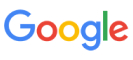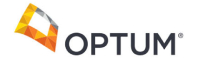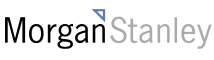+17 more companies

## Problem statement

You have been given a Binary Tree of 'N'

nodes, where the nodes have integer values.

Your task is to return the ln-Order, Pre-Order, and Post-Order traversals of the given binary tree.

For example :
``````For the given binary tree:
``````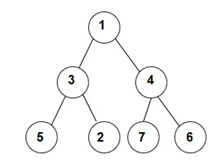``````The Inorder traversal will be [5, 3, 2, 1, 7, 4, 6].
The Preorder traversal will be [1, 3, 5, 2, 4, 7, 6].
The Postorder traversal will be [5, 2, 3, 7, 6, 4, 1].
``````
Detailed explanation ( Input/output format, Notes, Images )
Sample Input 1 :
``````1 2 3 -1 -1 -1  6 -1 -1
``````
Sample Output 1 :
``````2 1 3 6
1 2 3 6
2 6 3 1
``````
Explanation of Sample Output 1 :
`````` The given binary tree is shown below:
``````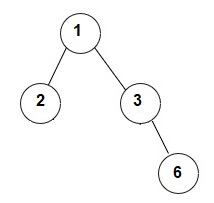``````Inorder traversal of given tree = [2, 1, 3, 6]
Preorder traversal of given tree = [1, 2, 3, 6]
Postorder traversal of given tree = [2, 6, 3, 1]
``````
Sample Input 2 :
``````1 2 4 5 3 -1 -1 -1 -1 -1 -1
``````
Sample Output 2 :
``````5 2 3 1 4
1 2 5 3 4
5 3 2 4 1
``````
Explanation of Sample Output 2 :
``````The given binary tree is shown below:
``````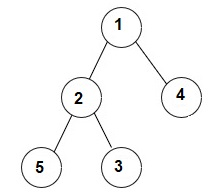``````Inorder traversal of given tree = [5, 2, 3, 1, 4]
Preorder traversal of given tree = [1, 2, 5, 3, 4]
Postorder traversal of given tree = [5, 3, 2, 4, 1]
``````
Constraints :
``````1 <= 'N' <= 10^5
0 <= 'data' <= 10^5

where 'N' is the number of nodes and 'data' denotes the node value of the binary tree nodes.

Time limit: 1 sec
``````Console